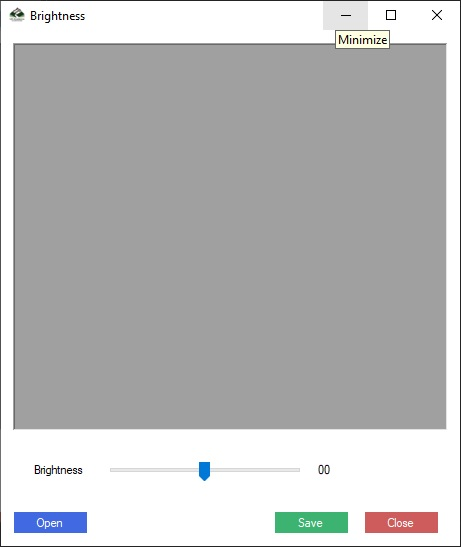# C#

This example shows a quick way to adjust the Brightness of an image

The idea is easy: move the red, green and blue colors of every pixel closer to the lowest or highest values 0 and 255. We make the pixels darker, for example if the component of a pixel is 128, 32, 254, and we change it to 64, 16, 127.

We can loop through pixels of an image and individually modify them, But a much quicker technique is available: draw the picture into a fresh Bitmap with the item ImageAttributes to adjust values of pixels.

In this manner, colors are represented by a vector with 5 entries when transforming colors. The first four of them are for the pixel parts: red, green, blue and alpha (opacity). The fifth entry consists of a value of scaling 1.A ColorMatrix object can convert colors of an image through a 5-by-5 matrix of floating point values to multiply each pixel's color vector.

Every color is left unchanged in the identity matrix, 1 is diagonals down, and 0 is everywhere.

For the red, green and blue components the following matrix applies the scaling factor S.

 S 0 0 0 0 0 S 0 0 0 0 0 S 0 0 0 0 0 S 0 0 0 0 0 S

The resulting matrix will be [ S*r, S*g, S*b, a, 1 ] when multiplied by the generic pixel color vector [ r, g, b, a,1 ] so the matrix can scale up the color components as desired.

The AdjustBrightness technique shown under the following code utilizes an item from ImageAttributes to use the ColorMatrix scaling on the picture pixels.

Code

``````
private Bitmap AdjustBrightness(Image image, float brightness)
{
// Make the ColorMatrix.
float b = brightness;
ColorMatrix cm = new ColorMatrix(new float[][]
{
new float[] {b, 0, 0, 0, 0},
new float[] {0, b, 0, 0, 0},
new float[] {0, 0, b, 0, 0},
new float[] {0, 0, 0, 1, 0},
new float[] {0, 0, 0, 0, 1},
});
ImageAttributes attributes = new ImageAttributes();
attributes.SetColorMatrix(cm);

// Draw the image onto the new bitmap while applying the new ColorMatrix.
Point[] points =
{
new Point(0, 0),
new Point(image.Width, 0),
new Point(0, image.Height),
};
Rectangle rect = new Rectangle(0, 0, image.Width, image.Height);

// Make the result bitmap.
Bitmap bm = new Bitmap(image.Width, image.Height);
using (Graphics gr = Graphics.FromImage(bm))
{
gr.DrawImage(image, points, rect, GraphicsUnit.Pixel, attributes);
}

// Return the result.
return bm;
}

``````

The code starts by creating an object ColorMatrix which scales the components of the pixel color red, green and blue.(We use variable b instead of brightness so that the columns of the matrix line up and facilitate reading.) Then the program creates an ImageAttributes object and uses its SetColorMatrix method to give it the ColorMatrix.

Next, a Point array and a rectangle are defined to show that the whole picture in the output region should be drawn and its initial size.(Some overloaded versions of the DrawImage method of Graphics objects take simple parameters, but do not allow you to use an object of ImageAttributes.)

The program creates a bitmap and a graphics object associated to it. The Graph element is used to draw the picture into the bitmap, passing the DrawImage method the ImageAttributes object. DrawImage uses the ColorMatrix object ImageAttributes to transform each pixel it draws, thus making the result brightness scaled.

This is extremely fast so you can pull back and forth the track bar to adjust the brightness in real time.Complete Source Code of Image Brightness In C#

``````
using System;
using System.Drawing;
using System.Drawing.Imaging;
using System.Windows.Forms;

namespace BrightnessContrast
{
public partial class frmBrightness : Form
{

Bitmap bitmap;
public frmBrightness()
{
InitializeComponent();
}

private void BtnSave_Click(object sender, EventArgs e)
{
picBox.Image.Save("output.jpg");
}

private void BtnClose_Click(object sender, EventArgs e)
{
Close();
}

private void BtnOpenImage_Click(object sender, EventArgs e)
{
if (DialogResult.OK == openFileDialog.ShowDialog())
{
bitmap = (Bitmap)Bitmap.FromFile(openFileDialog.FileName);
picBox.Image = bitmap;
}
}

private void TraBrightness_Scroll(object sender, EventArgs e)
{
lblBrightnessValue.Text = "Brightness = " + (traBrightness.Value / 100.0).ToString();
picBox.Refresh();
}
private Bitmap AdjustBrightness(Image image, float brightness)
{
// Make the ColorMatrix.
float b = brightness;
ColorMatrix cm = new ColorMatrix(new float[][]
{
new float[] {b, 0, 0, 0, 0},
new float[] {0, b, 0, 0, 0},
new float[] {0, 0, b, 0, 0},
new float[] {0, 0, 0, 1, 0},
new float[] {0, 0, 0, 0, 1},
});
ImageAttributes attributes = new ImageAttributes();
attributes.SetColorMatrix(cm);

// Draw the image onto the new bitmap while applying the new ColorMatrix.
Point[] points =
{
new Point(0, 0),
new Point(image.Width, 0),
new Point(0, image.Height),
};
Rectangle rect = new Rectangle(0, 0, image.Width, image.Height);

// Make the result bitmap.
Bitmap bm = new Bitmap(image.Width, image.Height);
using (Graphics gr = Graphics.FromImage(bm))
{
gr.DrawImage(image, points, rect, GraphicsUnit.Pixel, attributes);
}

// Return the result.
return bm;
}
}
}

``````